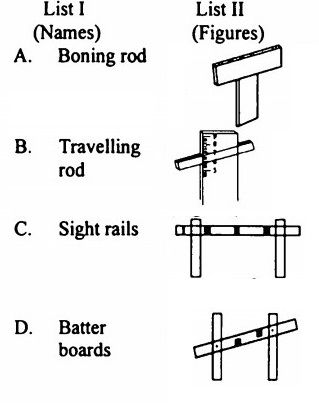top of pageSearch

# Survey (341-360)

Updated: Apr 25, 2020

341. Given that

f = focal length of camera,

H = height of exposure station above MSL and

h = height of ground above MSL.

The scale of vertical photograph is given by

S = f/(H-h)

342. The main plate of a transit is divided into 1080 equal divisions. 60 divisions of the vernier coincide exactly with 59 divisions of the main plate. The least count (in

seconds) of the transit is 20

343. The sides of a rectangle are (120 ± 0.05) m and (180 ± 0.06) m. The probable error in the area will be ±11.53 sqm

344. The linear error in a 50 m long traverse is 0.01 rtK The angular error (in seconds) considering equal precision will be 41

345. A 30 m long steel tape standardised with a pull of 100 N was used for measuring a baseline of length 1500 m. The pull exerted while measuring is 150 N. The. correction Cp due to pull is given by (the area of cross-section of the tape = A;

Young's modulus = E) 50 x 1500/AE

346. Mean sea level at any place is the average datum of hourly tide heights observed over a period of nearly 20 years

347. Excavation is to be made for a reservoir measuring 20 m long, 12 m wide at the bottom and 2 m deep. The side slopes are to be 1 : 1 and the top to be flush with the ground which is level in the vicinity. As per prismoidal formula, the volume of excavation will be 618.66 m³

348. Given that for a planimeter

L = length of the tracing arm

R = radius of the anchor arm

a = distance between the roller and hinge

If the wheel is beyond the hinge, then the area of zero circle will be

π(L² + 2aL + R²)

349. For a, sight inclined at θ° with the horizontal and the staff held normal to the

line of sight, the staff intercept is S. The horizontal distance D between the

tacheometer attached with an anallatic

(kS + c) cos θ + r sin θ

350. Match List I with List II and select the correct answer using the codes given

below the Lists351. Given that for a triangulation survey

D = distance in km

h = the visible horizon from a station of known elevation above the datum (in

metres)

If there is no obstruction due to intervening ground, then h is equal to

0.0673 D²

352. For solving a right-angled astronomical triangle ZPS, right-angled at S. the parts

of the Napter circle are to be filled up by the following elements:

1. The sides PS and ZS

2. Complement of the angle SZP.

3. Complement of the side ZP

4. Complement of the angle ZPS

The correct sequence of filling up these

elements is

353A. Star at elongation-When it is at its greatest distance eas or west of meridian B. Star at prime vertical-The astronomical triangle is evidently right-angled at zenith C. Star at horizon-Its altitude will be zero and zenith distance will be equal to 90° D. Star at culmination-When it crosses the observer's meridian

354. The latitude and longitude of point M is 30° N and 15° W. The latitude and

longitude of point N is 20° S and 25° E. MN will be given by

- cos 60° cos 70° + sin 60° sin 70° cos 40

355.A.Principal point-The point where a perpendicular dropped from the front nodal point strikes the photograph B. Isocentre-The point at which the bisector of the angle of tilt meets the photographer C. Crab-The angle formed between the flight line and the edges of photograph in the direction of flight D. Drift-Failure of the aeroplane to stay on the predetermined flight line

356. Given that Scale of the photograph, 50 m = 1 cm Size of photograph = 18 cm x 18 cm Percentage longitudinal overlap = 60 Percentage side overlap = 33 1/3 Covered area = 108 sq km The required number of photographs will be

500

357. Images of two objects on a pair of photo graphs have a parallax difference of 1.8 mm and an average photograph base of 88.2 mm. The flying height is 4000 m

above the average ground level. The difference in elevation of the two objects

would be. 80 m

358. The given table shows the bearings observed while traversing with a compass:Which one of the following pairs of stations is affected by local attraction?

B and C

a) Both A and R are true and R is the correct explanation of A

b) Both A and R are true but R is not a correct explanation of A

c) A is true but R is false

d) A isfalse but R is true

359. Assertion A : Nautical sextant is used in hydrographic surveying.

Reason R : This instrument helps in determining the depth of water.

a

360. Assertion A : Rise and fall method is preferred for reduction of levels in

important works.

Reason R : It provides a complete check on reduction of levels of all points.

a